# Grouping and Aggregating with Pandas

• Last Updated : 15 Mar, 2021

In this article, we are going to see grouping and aggregating using pandas. Grouping and aggregating will help to achieve data analysis easily using various functions. These methods will help us to the group and summarize our data and make complex analysis comparatively easy.

Creating a sample dataset of marks of various subjects.

## Python

 `# import module``import` `pandas as pd`` ` `# Creating our dataset``df ``=` `pd.DataFrame([[``9``, ``4``, ``8``, ``9``],``                   ``[``8``, ``10``, ``7``, ``6``],``                   ``[``7``, ``6``, ``8``, ``5``]],``                  ``columns``=``[``'Maths'``,  ``'English'``, ``                           ``'Science'``, ``'History'``])`` ` `# display dataset``print``(df)`

Output: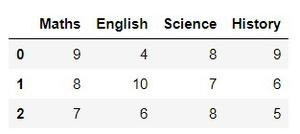## Aggregation in Pandas

Aggregation in pandas provides various functions that perform a mathematical or logical operation on our dataset and returns a summary of that function. Aggregation can be used to get a summary of columns in our dataset like getting sum, minimum, maximum, etc. from a particular column of our dataset. The function used for aggregation is agg(), the parameter is the function we want to perform.

Some functions used in the aggregation are:

Function Description:

• sum()         :Compute sum of column values
• min()          :Compute min of column values
• max()         :Compute max of column values
• mean()       :Compute mean of column
• size()          :Compute column sizes
• describe()  :Generates descriptive statistics
• first()          :Compute first of group values
• last()          :Compute last of group values
• count()       :Compute count of column values
• std()           :Standard deviation of column
• var()           :Compute variance of column
• sem()         :Standard error of the mean of column

Examples:

• The sum() function is used to calculate the sum of every value.

## Python

 `df.``sum``()`

Output: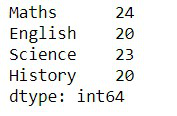• The describe() function is used to get a summary of our dataset

## Python

 `df.describe()`

Output: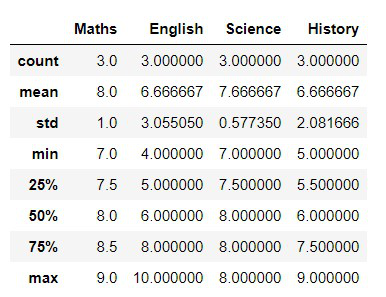• We used agg() function to calculate the sum, min, and max of each column in our dataset.

## Python

 `df.agg([``'sum'``, ``'min'``, ``'max'``])`

Output: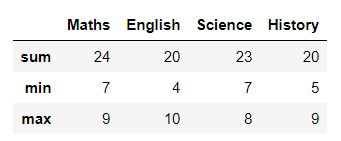## Grouping in Pandas

Grouping is used to group data using some criteria from our dataset. It is used as split-apply-combine strategy.

• Splitting the data into groups based on some criteria.
• Applying a function to each group independently.
• Combining the results into a data structure.

Examples:

We use groupby() function to group the data on “Maths” value. It returns the object as result.

## Python

 `df.groupby(by``=``[``'Maths'``])`

Output:

`<pandas.core.groupby.generic.DataFrameGroupBy object at 0x0000012581821388>`

Applying groupby() function to group the data on “Maths” value. To view result of formed groups use first() function.

## Python

 `a ``=` `df.groupby(``'Maths'``)``a.first()`

Output: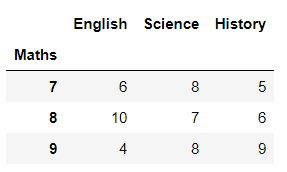First grouping based on “Maths” within each team we are grouping based on “Science”

## Python

 `b ``=` `df.groupby([``'Maths'``, ``'Science'``])``b.first()`

Output: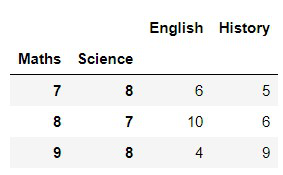## Implementation on a Dataset

Here we are using a dataset of diamond information.

## Python

 `# import module``import` `numpy as np``import` `pandas as pd`` ` `# reading csv file``dataset ``=` `pd.read_csv(``"diamonds.csv"``)`` ` `# printing first 5 rows``print``(dataset.head(``5``))`

Output: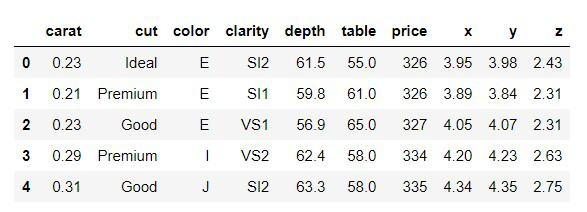• We group by using cut and get the sum of all columns.

## Python

 `dataset.groupby(``'cut'``).``sum``()`

Output: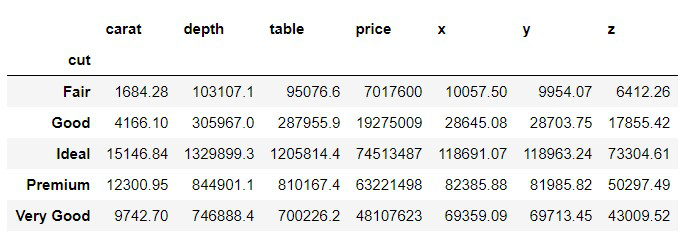• Here we are grouping using cut and color and getting minimum value for all other groups.

## Python

 `dataset.groupby([``'cut'``, ``'color'``]).agg(``'min'``)`

Output: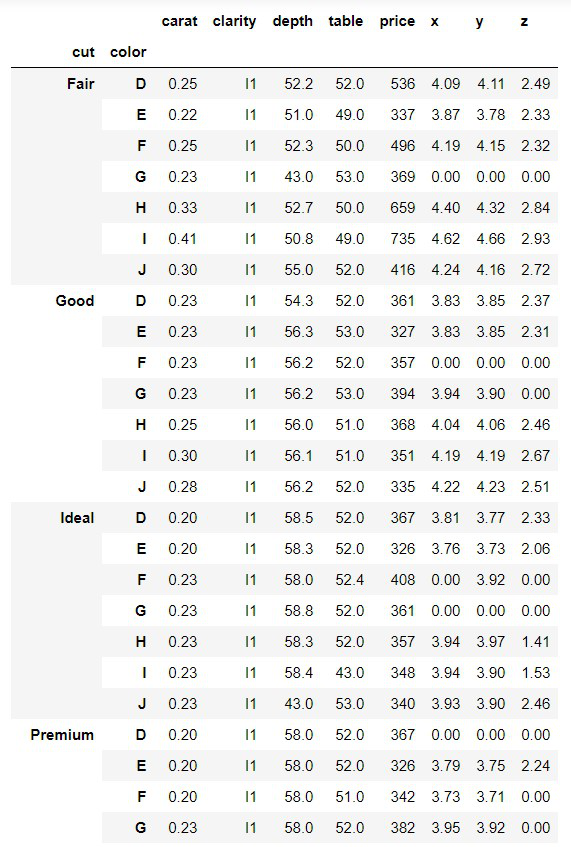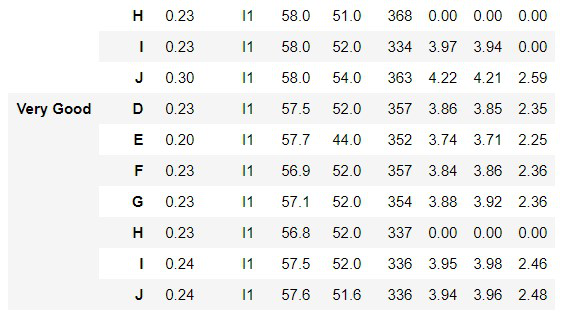• Here we are grouping using color and getting aggregate values like sum, mean, min, etc. for the price group.

## Python

 `# dictionary having key as group name of price and``# value as list of aggregation function ``# we want to perform on group price``agg_functions ``=` `{``    ``'price'``:``    ``[``'sum'``, ``'mean'``, ``'median'``, ``'min'``, ``'max'``, ``'prod'``]``}`` ` `dataset.groupby([``'color'``]).agg(agg_functions)`

Output: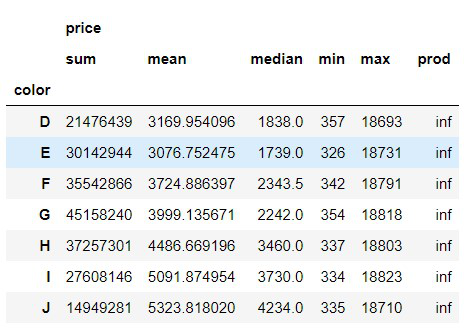We can see that in the prod(product i.e. multiplication) column all values are inf, inf is the result of a numerical calculation that is mathematically infinite.

My Personal Notes arrow_drop_up